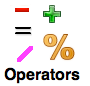# OperatorsIn this lesson you will be introduce to these operators
→ Arithmetic operators
→ Logical operators
→ Relational operator
→ The conditional (ternary) operator

### Arithmetic Operators

These arithmetic operators let you perform basic math operations.

Operator Operation Example
+ Addition sum = numOne + numTwo;
Substraction sum = 17-10
/ Division  result = 25 / 5;
* Multiplication sum = 10*3;
% Modulus The Modulus operator, returns the integer remainder after division. So the number 1 is assign in the remainder variable.
remainder = 10 % 3
++ Increment Increment the counter variable by 1
int counter=0;counter++; // counter is now equal to 1
Decrement Decrement the counter variable by 1
int counter=13; counter–;  // counter is now equal to 12
+= Compound assignment float balance=100.00; balance += 500.00;  // balance now contain 600
-= Compound decrement float balance = 750; balance -= 500;  // balance now contain 250
*= Compound multiplication float balance = 15000.00;
balance *= 2;  // balance now contain 30000.00
/= Compound division float balance = 40000.00;
abalance /= 2;  // balance now contain 20000.00

### Logical Operators

These logical operators connect two or more relational expressions into one or reverse the logic of an expression.

Operator Description
! This is the NOT operator. It reverses the “truth” of an expression. It makes a true expression false, and a false expression true. Here is the operator’s truth table.

Example of Use

```float hoursWorked = 26.50;
if (!(hoursWorked > 40))
outputBox.text = @"You don't qualify for overtime pay.";
```
&& This is the AND operator. It connects two expressions into one. Both expressions must be true for the overall expression to be true.
Here is the operator’s truth table. The table lists all the possible combinations of values that two expressions may have, and the resulting value returned by the && operator connecting the two expressions.

Example of Use

```char employed = 'Y', recentGrad = 'Y';
if (employed == 'Y' && recentGrad == 'Y')
NSLog(@"You qualify for the special interest rate.");
```
|| This is the OR operator. It connects two expressions into one. One or both expressions must be true for the overall expression to be true. It is only necessary for one to be true, and it does not matter which one. Here is the operator’s truth table. The table lists all the possible combinations of values that two expressions may have, and the resulting value returned by the || operator connecting the two expressions.

Example of Use

```char raining = 'Y', sunny = 'Y';
if (raining == 'Y' || sunny == 'Y')
NSLog(@"Take your umbrella when you go outside today.");
```

### Relational Operators

These relational operators allow you to compare two numeric values or two char values. The type of the result is int and has the values 1 if the specified relationship is true, and 0 if false. This table list C’s relational operators.

Operator Meaning Usage Example
Assume numOne holds 25 and numTwo holds 10
> Greater than // true
numOne > numTwo;
< Less than // false
numOne < numTwo;
>= Greater than or equal to // true
numOne >= numTwo;
<= Less than or equal to [// false
numOne <= numTwo;
== Equal to // false
numOne == numTwo;
!= Not equal to // true
numOne != numTwo;

#### The Conditional (Ternary) Operator

The conditional operator is a simpler alternative to setting a variable using an if/else conditional statement. We can take the following if/else conditional statement:

```char lightBulbOn = 'n';
if (lightBulbOn == 'y')
NSLog(@"The light bulb is on.");
else
NSLog(@"The light bulb is off.");
```

And replace it with this conditional operator:

```// The parentheses around the conditional statement are not required. I'm using them as a visual guide
outputBox.text = (lightBulbOn == 'y') ? @"The light bulb is on." : @"The light bulb is off.";
```

Syntax
variable = (condition ) ? true : false;

If the value of the lightBulbOn variable equals ‘y’, then the outputBox.text control is set to “The light bulb is on”. Otherwise, it is set to “The light bulb is off.”. Once you grasp how the conditional (ternary) operator works it is a great tool to use to trim down your code and simplify your conditional statement.